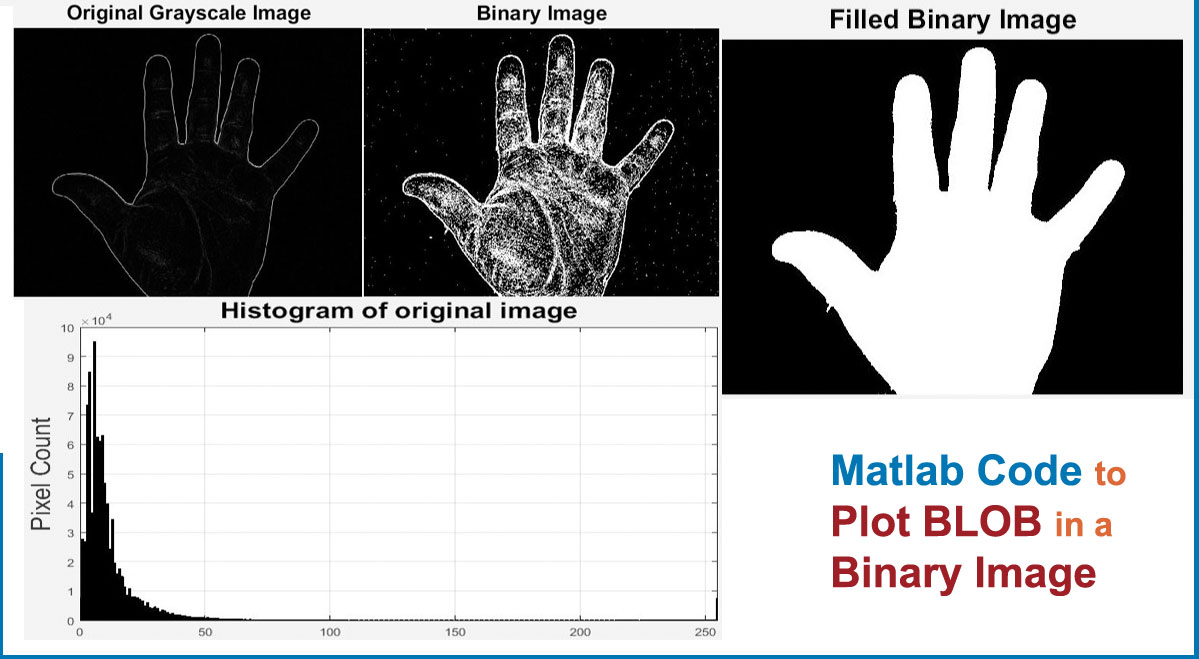## Matlab Code to Plot BLOB in a Binary Image

• Post author:
• Post category:Uncategorized

Blob are binary large object in Image,Blobs are generally used in image and video processing for many applications like,Vehicle tracking,People Counting,Background subtraction ,Gait recognition and much more.

This program explains to plot the boundaries of a blob in binary images.

```clc;
clear;
close all;

%Convert Image to Binary
BW=im2bw(BW);

% Trace region boundaries in a binary image.
[B,L,N,A] = bwboundaries(BW);

%Display Images
imshow(BW); hold on;

for k=1:length(B),

if(~sum(A(k,:)))

boundary = B{k};

plot(boundary(:,2), boundary(:,1), 'r','LineWidth',2);

for l=find(A(:,k))'

boundary = B{l};

plot(boundary(:,2), boundary(:,1), 'g','LineWidth',2);

end

end

end

```